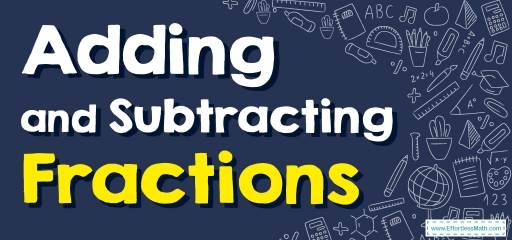# How to Add and Subtract Fractions? (+FREE Worksheet!)

The fraction is a part of a whole or, more generally, any number of equal components. In everyday conversation, fractions are used to describe how many components there are with certain values.Each fraction consists of a numerator that is placed above the line and a non-zero denominator that is placed below that line. In this post, you can learn how to add and subtract fractions in a few easy steps.

## Related Topics

The Absolute Best Book for 4th Grade Students

## Step-by-step guide for Adding and Subtracting Fractions

Addition and subtraction of fractions are of two types:

1- Addition and subtraction of “like” fractions with the same denominator:

• For “like” fractions, if the denominator of all fractions is the same, the numerators of all fractions are added together. In this case, the denominator does not change and you can write the answer over the common denominator.

2- Addition and subtraction of “unlike” fractions with different denominators:

• Step 1: Find equivalent fractions with the same denominator before you add or subtract fractions with different denominators. Adding and Subtracting with the same denominator:
$$\frac{a}{\color{blue}{b}} \ + \ \frac{c}{ \color{ blue }{b} } = \frac{a \ + \ c}{ \color{ blue }{b} }$$ , $$\frac{a}{ \color{ blue }{b} } \ – \ \frac{c}{ \color{ blue }{b} } =\frac{a \ – \ c}{ \color{ blue }{b} }$$
• Step 2: Multiply two denominators (or as many as there are) to get a common denominator.
• Step 3:  If one of the denominators was divisible by the other denominators, select the greater denominator as the common denominator.
• Step 4: The numerators are enlarged to fit the common denominator. In this case, the numerators of fractions are added together.
$$\frac{a}{ \color{red}{b} } \ + \ \frac{c}{ \color{blue}{d} } = \frac{a \color{blue}{d} \ + \ \color{red}{b} c}{ \color{red}{b} \color{blue}{d} }$$ , $$\frac{a}{ \color{red}{b} } \ – \ \frac{c}{ \color{blue}{d} }=\frac{a \color{blue}{d} \ – \ c \color{red}{b} }{ \color{red}{b} \color{blue}{d} }$$

### Adding and Subtracting Fractions – Example 1:

Subtract fractions. $$\frac{4}{6} \ – \ \frac{3}{6} =$$

Solution:

For “like” fractions, subtract the numerators and write the answer over the common denominator. then: $$\frac{4}{6} \ – \ \frac{3}{6}=\frac{4 \ – \ 3}{6}=\frac{1}{6}$$

A Perfect Book for Grade 4 Math Word Problems!

### Adding and Subtracting Fractions – Example 2:

Add fractions. $$\frac{3}{7} \ + \ \frac{2}{3}=$$

Solution:

For “unlike” fractions, find equivalent fractions with the same denominator before you can add or subtract fractions with different denominators. Use this formula: $$\frac{a}{\color{red}{b}} \ + \ \frac {c}{\color{blue}{d}}=\frac{a \color{blue}{d} \ + \ c \color{red}{b} }{ \color{red}{b} \color{blue}{d} }$$
$$\frac{3}{\color{red}{7}} \ + \ \frac{2}{\color{blue}{3}}=\frac{(3)( \color{blue}{3} ) \ + \ (2)( \color{red}{7} )}{ \color{red}{7} \ \times \ \color{blue}{3} }=\frac{9 \ + \ 14}{21}=\frac{23}{21}$$

### Adding and Subtracting Fractions – Example 3:

Subtract fractions. $$\frac{4}{5} \ – \ \frac{3}{5}=$$

Solution:

For “like” fractions, subtract the numerators and write the answer over the common denominator. then: $$\frac{4}{5}-\frac{3}{5}= \frac{4 \ – \ 3}{5}= \frac{1}{5 }$$

### Adding and Subtracting Fractions – Example 4:

Subtract fractions. $$\frac{2}{3} \ – \ \frac{1}{2}=$$

Solution:

For “unlike” fractions, find equivalent fractions with the same denominator before you can add or subtract fractions with different denominators. Use this formula: $$\frac{a}{ \color{red}{b} } \ – \ \frac{c}{ \color{blue}{d} }=\frac{a \color{blue}{d} \ – \ c \color{red}{b} }{ \color{red}{b} \color{blue}{d} }$$
$$\frac{2}{\color{red}{3}} \ – \ \frac{1}{\color{blue}{2}}=\frac{(2)( \color{blue}{2} ) \ – \ (1)( \color{red}{3} )}{ \color{red}{3} \ \times \ \color{blue}{2} }=\frac{4 \ – \ 3}{6}=\frac{1}{6}$$

## Exercises for Adding and Subtracting Fractions

### Add fractions and Subtract fractions.

1. $$\color{blue}{\frac{2}{3}+\frac{1}{2}}$$
2. $$\color{blue}{\frac{3}{5}+\frac{1}{3}}$$
3. $$\color{blue}{\frac{5}{6}+\frac{1}{2}}$$
4. $$\color{blue}{\frac{4}{5}-\frac{2}{5}}$$
5. $$\color{blue}{\frac{3}{5}-\frac{2}{7}}$$
6. $$\color{blue}{\frac{1}{2}-\frac{1}{3}}$$

1. $$\color{blue}{\frac{7}{6}}$$
2. $$\color{blue}{\frac{14}{15}}$$
3. $$\color{blue}{\frac{4}{3}}$$
4. $$\color{blue}{\frac{2}{5}}$$
5. $$\color{blue}{\frac{11}{35}}$$
6. $$\color{blue}{\frac{1}{6}}$$

The Best Math Books for Elementary Students

### What people say about "How to Add and Subtract Fractions? (+FREE Worksheet!) - Effortless Math: We Help Students Learn to LOVE Mathematics"?

No one replied yet.

X
51% OFF

Limited time only!

Save Over 51%

SAVE $15 It was$29.99 now it is \$14.99